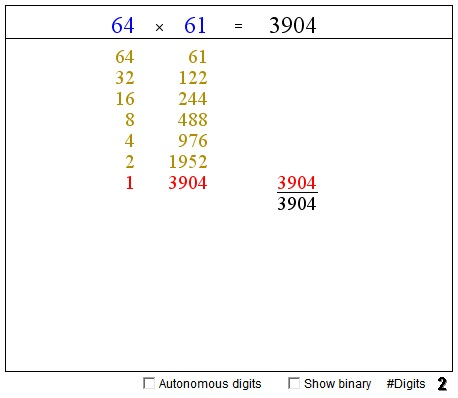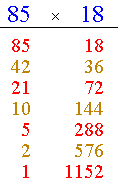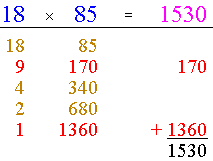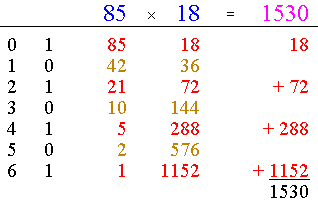# Peasant Multiplication

The multiplication algorithm [Wells, p. 44] discussed below is commonly known as the Russian Peasant Multiplication. It is even said that the algorithm "is still used by peasants in some areas, such as Russia." However, the source of the Russian Peasant designation is unexpectedly murky. It probably goes back to a few centuries old Russian book where the method has been first described in (relatively) modern times. I may only conjecture that the algorithm has acquired the Russian part of the designation in the process of translation from Russian and the Peasant part was appended due to a widely spread conviction that (at least in older times) it was mostly the peasant population that exclusively, albeit sparsely, filled the Russian vastness.

The algorithm in fact may have Egyptian roots, as a similar procedure has been routinely used in the famous Rhind Papyrus [Midonick, pp. 706-732, Fauvel, pp. 14-16]. It is sometimes referred to as the Ethiopian (Peasant) Multiplication; the linkage could be explained by the proximity of the two nations and intermixing of their cultures. It is curious to note in passing that the great-grandfather of the illustrious Russian poet Alexander Serge'evich Pushkin was a blackamoor of Ethiopian origin. However, the spurious idea that Ibrahim Petrovitch Gannibal, a page to Peter the Great, may be a historic conduit for the algorithm from North Africa to Russia clashes with the Peasant part of the designation. A pity.

The applet below allows for experimentation with the algorithm I'll present shortly. The two blue numbers at the top - the multiplicands - can be modified by clicking on their digits. (The digits can be treated individually or as part of a number depending on the state of the "Autonomous digits" checkbox.) The number of digits in the mutiplcands changes from 1 through 4.

### If you are reading this, your browser is not set to run Java applets. Try IE11 or Safari and declare the site https://www.cut-the-knot.org as trusted in the Java setup.What if applet does not run?

The algorithm instructs us to create a column beneath each of the multiplicands. We start by dividing the first number by 2 (and dropping the remainder if any) and recording the result in the first column. Then we divide by 2 the recorded number and write the result below. The process of division by two of the successive results continues until 1 is reached. In the second column we write the numbers obtained by successive multiplication by 2 that starts with the second multiplicand. Let's see how it works for the product 85×18:

Dividing 85 by 2 (and dropping the remainder) we get successively: 85, 42, 21, 10, 5, 2, 1. Now, multiply by 2 the second number, 18, as many times: 18, 36, 72, 144, 288, 576, 1152. Let's write these in two columns:I listed the numbers in two colors for an important reason. We have to look at the first column and mark there the odd numbers. These appear in red. The even numbers are orange - diluted red - to signify their lack of importance for the next step. On the next step, mark the numbers in the second column that are in the same rows with the odd numbers in the first. These are also in red. For the convenience sake, I added a third column where the red numbers from the second one have been separated:All that remains at this stage is to add up all the numbers in the third column. The result is 1530, exactly the product 85×18. You can check that the algorithm yields the same result when applied to the product 18×85:As you can see, the result is the same, but is obtained in fewer steps. This is true in general: if tasked with applying the algorithm to finding the product of two numbers a and b, make the smaller number first, the larger one second.

Now, let's see why the algorithm works. Since halving and doubling play such an important role in the algorithm, it should not come as a great surprise that its real foundation lies in the binary system. To obtain the binary representation of a number, the number is to be repeatedly divided by two, the remainders recorded and then written in the reverse order. Check now the "Show binary" box:We see two additional columns: the first indicates the step (and a power of 2), the second contains the binary digits of 85 written from the bottom upwards:

85 = 10101012,

which essentially means that

 85 = 10101012 = 1·26 + 0·25 + 1·24 + 0·23 + 1·22 + 0·21 + 1·20 = 64 + 16 + 4 + 1.

Note that a binary digit is the remainder of division by 2: it is 1 for odd numbers and 0 for even numbers. Which bring forth the connection between the binary digits of the first multiplicand, 85 in our case, and the parity of the number in the column beneath it. The numbers are odd exactly when the remainder that appears to their left is 1. This makes the algorithm tick:

 85×18 = (64 + 16 + 4 + 1)×18 = 1152 + 288 + 72 + 18 = 1530.

For the product 18×85 we get:

 18 = 100102 = 1·24 + 0·23 + 0·22 + 1·21 + 0·20 = 16 + 2.

and subsequently

 18×85 = (16 + 2)×85 = 1360 + 170 = 1530.

### References

1. J. Fauvel, J. Gray (eds), The History of Mathematics. A Reader, The Open University, 1987
2. H. O. Midonick (ed), The Treasury of Mathematics, Philosophical Library, 1965
3. D. Wells, The Penguin Dictionary of Curious and Interesting Numbers, Penguin Books, 1987.•